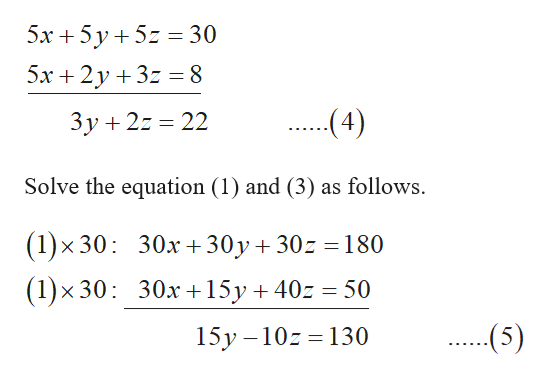# Solve using eliminationx + y + z= 65x + 2y + 3z =86x + 3y + 8z = 10

Question
2 views

Solve using elimination

x + y + z= 6

5x + 2y + 3z =8

6x + 3y + 8z = 10

check_circle

Step 1

The equations are given by,

Step 2

Multiply the equation (1) by 5 and subtr...help_outlineImage Transcriptionclose5х + 5у+52 %3D30 5x 2y3z = 8 ...4) Зу+ 22 — 22 Solve the equation (1) and (3) as follows. (1)x 30: 30х+ 30у + 302 %3D180 (1)x 30 30x 15y 40z 50 (5) 15y -10z 130 fullscreen

### Want to see the full answer?

See Solution

#### Want to see this answer and more?

Solutions are written by subject experts who are available 24/7. Questions are typically answered within 1 hour.*

See Solution
*Response times may vary by subject and question.
Tagged in

### Geometry